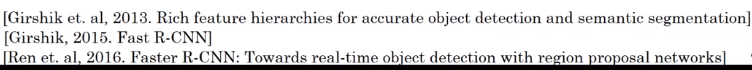# [Convolutional Neural Networks] week3. Object detection

### Object Localization

Classification VS. Localization VS. Detectionclassification with localization
Apart from softmax output (for classification), add 4 more outputs of bounding box: `b_x, b_y, b_h, b_w`.Defining target label y in localization
label format:
`P_c` indicating if there's any object
bounding box: `b_x, b_y, b_h, b_w`
class proba: `c_1, c_2, c_3`

Loss function: squared error
if y_1=P_c=1: loss = square error (y, y_hat)
if y_1=P_c=0: loss = (y_1 - y_1_hat)^2
can use different loss function for different components, but sq-loss works in practice.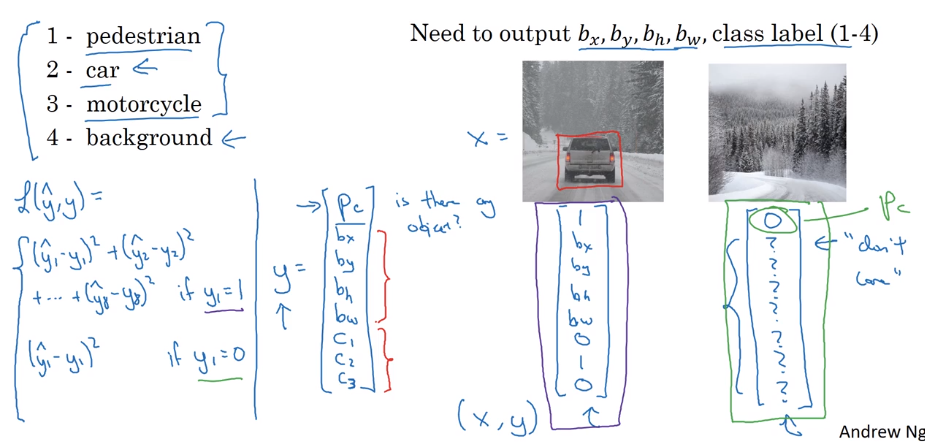### Landmark Detection

"landmark": important points in image. → let NN output their coords.

e.g. recognize coord of eye's corner or points along the eye/nose/mouth
→ specify a number of landmarks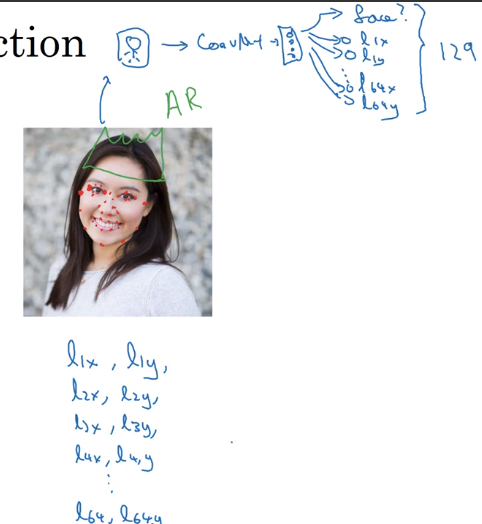### Object Detection

sliding windows detection
example: car detection.
training image: closely-croped image
in prediciton: use sliding window and pass to ConvNet; use window of different size.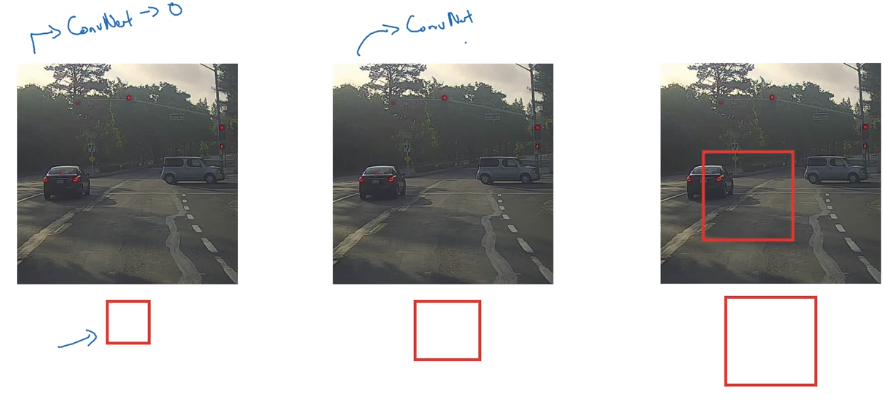Sliding window is OK with pre-DL algos.
disadvantage: computation cost too high — each window's crop ran independently through ConvNet.
→ sliding window also can be implemented "convolutionally" — some computation can be cached.

### Convolutional Implementation of Sliding Windows

Turning FC layer into conv layers
example: last conv/maxpool layer: size=55
replace FC(output_dim=400) by 400 55 filters
→ replace next FC layer by 11 filters
→ replace softmax layer by 1
1 filters and activation.conv implementation of sliding window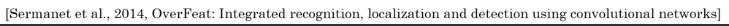example: training image 14143, testing image 16163
instead of corping image to 1414 and feed to ConvNet, feed the larger picture directly to ConvNet*.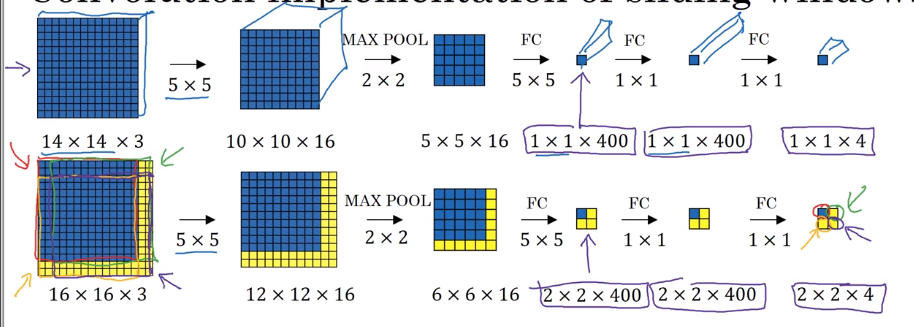output contains results of all patches!
⇒ instead of computing each sliding window sequentially, can get all results with a single pass of the full image!!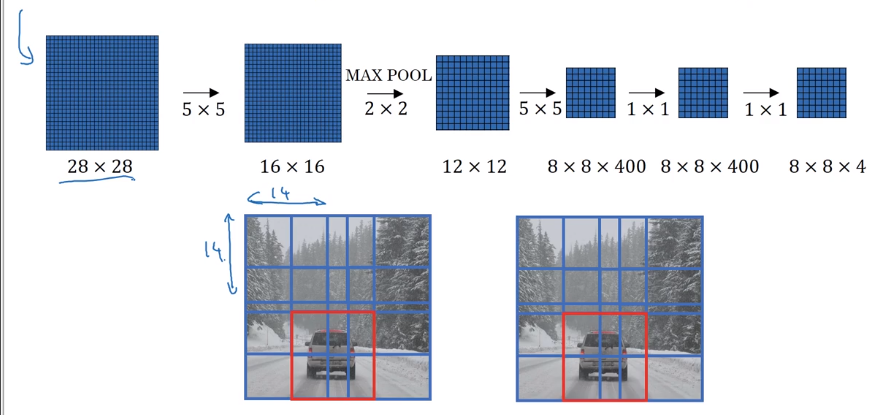problem: bounding box position is not accurate.

### Bounding Box Predictions

To output more accurate bounding boxes: aspect-ration no longer 1:1.
YOLO algorithm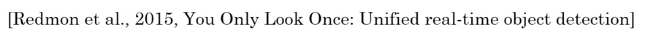"You Only Look Once"
For each grid cell: apply image classification with bouding boxes (described in 1st section, 8 outputs).
needs labelled data: assign each obj to the grid where its center is in.
output volume: 338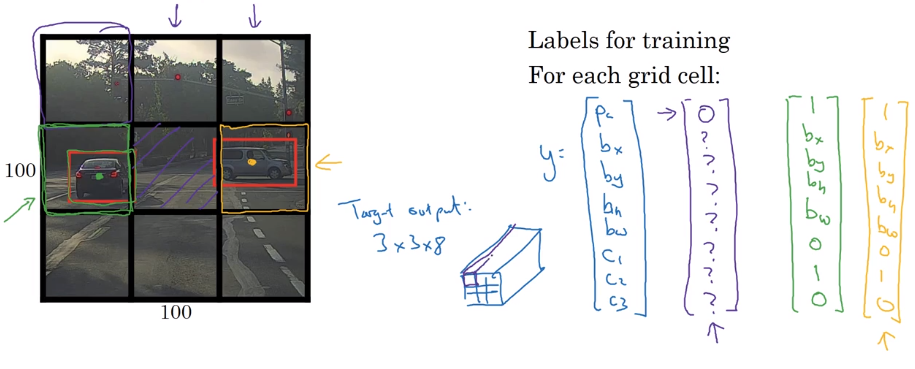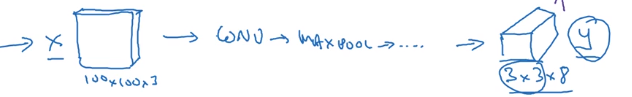Also: a lot of computation shared, efficient ⇒ possible to do real-time.

note: bounding box annotation in YOLO can be out of [0,1] range.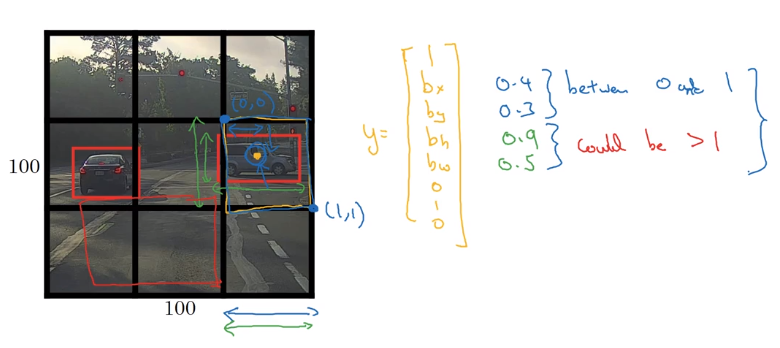### Intersection Over Union

Evaluating object localization:
→ intersection over union (IoU) function = size(intesection) / size(union) = measure of overlap of two bounding boxes.
"correct" if IoU >= 0.5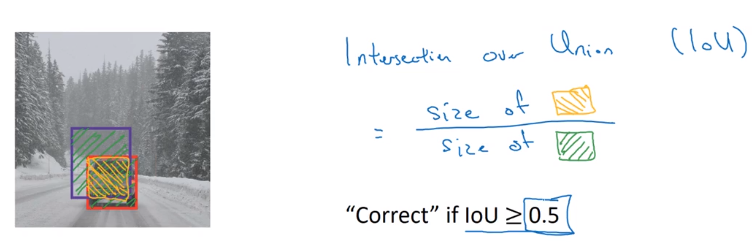### Non-max Suppression

Problem: algo might detect the same obj multiple times.
example: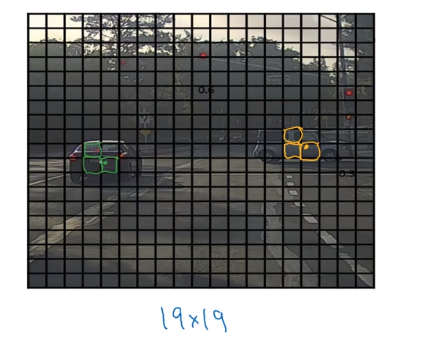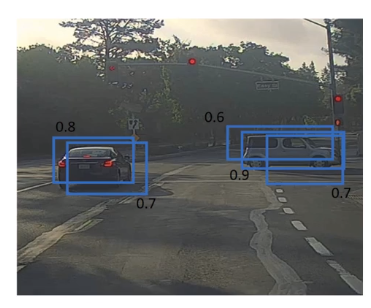each bouding box has a confidence score — keep the max bouding box, suppress the overlapping ones.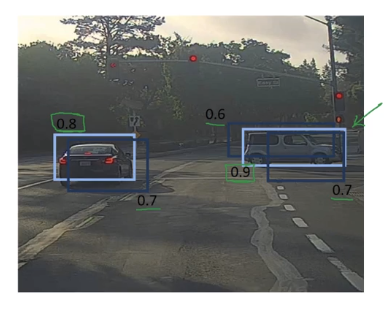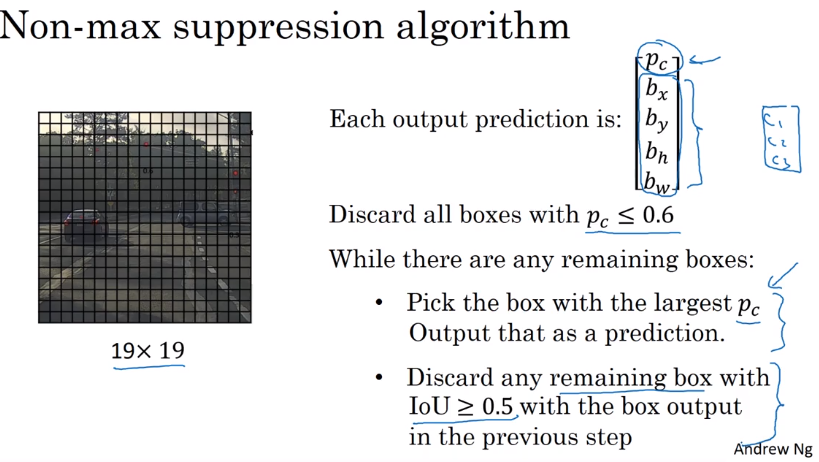### Anchor Boxes

Problem: each grid detects only one obj → can a grid detect multiple obj ? → use anchor boxes.

In data labeling: predefine 2 shapes (anchor boxes); use 8 sets of 8 outputs for each anchor box.Compare with previous:

• previous: each obj assigned to the grid which contains its mid point
• now each obj assigned to (cell, anchorbox): cell=the grid which contains its mid point; anchor_box= the anchorbox that has highest IoU with the labelled bounding box.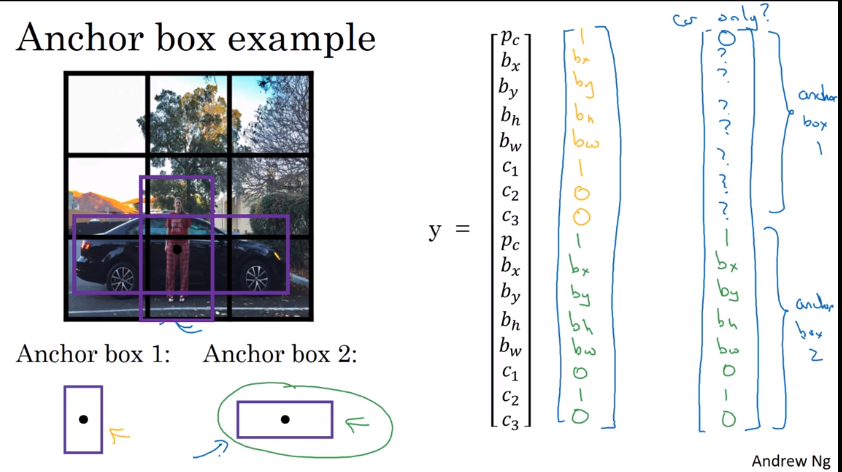In practice: choose 5~10 anchor boxes by hand; or use Kmeans on object's shapes.

### YOLO Algorithm

Put all components together.
example:

• detecting pedestrian/car/motercycle. (4 classes)
• grid: 3*3
• 2 anchor boxes

Preparing training set
y shape = 3*3*2*8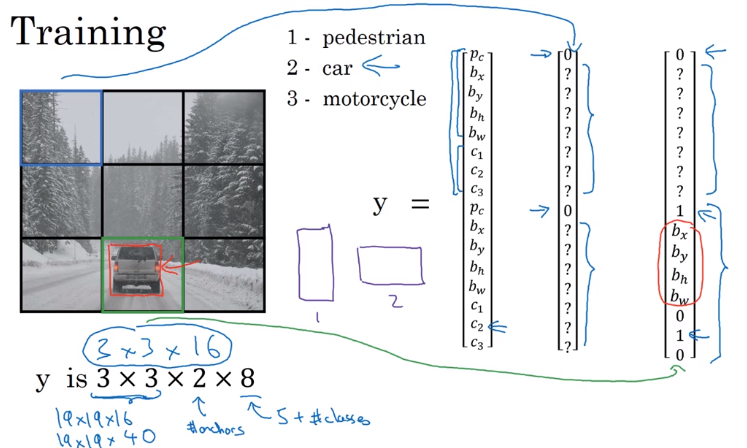train a ConvNet on this with output_dim = 3316
making predictions
2*8 outputs for each of the 9 grids
nonmax supression for each class### (Optional) Region ProposalsRegion proposal algo (R-CNN): less often than YOLO.
Sliding window disadvantage: many regions are not interesting.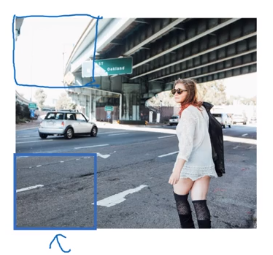⇒ select just a few windows
first run segmentation algo, then run CNN on bounding box of blobs.→ still quite slow
faster variants: